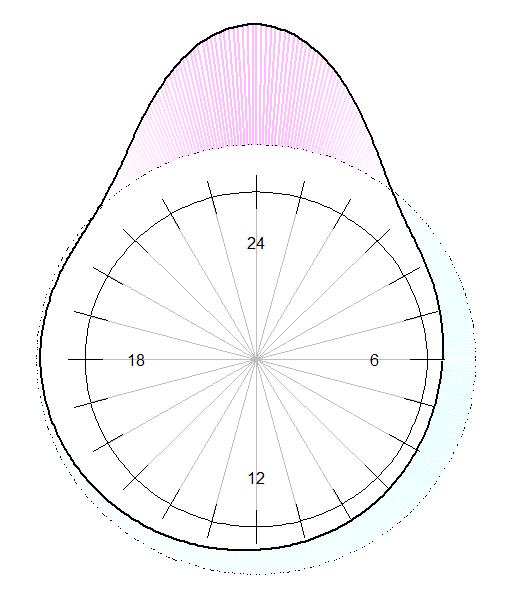Want to share your content on R-bloggers? click here if you have a blog, or here if you don't.

This afternoon, Jean-Luc asked me some help about an old post I did publish, minuit, l’heure du crime; and some graphs published a few days after, where I used a different visualisation, in another post.

The idea is that the hour can be seen as circular, in the sense that 23:58 is actually very close to 00:03. So when we use a nonparametric kernel estimator of time events, we have to take into account that property. More specifically, consider the density of an angle, i.e. a functionsuch thatwith a circular relationship, in the sense that.

In the dataset sent by Jean-Luc, we have some thefts in a big city, in France. The dataset is a simple spreadsheet with one columns, with ’00:20′ or ’17:45′ inside. Those are more or less reported time of thefts, as declared to the police.

B=read.table("Temp_Heures_VV.csv",header=TRUE,
sep=";")
HM=as.character(B[,1])
H=substr(HM,1,(nchar(HM)-3))
M=substr(HM,(nchar(HM)-1),(nchar(HM)))
X=as.numeric(H)+as.numeric(M)/60

The time is a number from 0 to 24.

U=seq(0,1,by=1/250)
O=U*2*pi
U12=seq(0,1,by=1/24)
O12=U12*2*pi
OM=2*pi*X/24
XL=c(X-24,X,X+24)
d=density(X)
d=density(XL,bw=d$bw,n=1500) I=which((d$x>=6)&(d$x<=30)) Od=d$x[I]/24*2*3.141592-3.141592/2
Dd=d$y[I]/max(d$y)+1

The idea to get a nice density estimation is to use a simple mirror technique : we have three versions of the data, one for today, one for yesterday, and one for tomorrow. Of course, we have to use a shorter bandwidth.

R=1/24/max(d$y)/3+1 plot(cos(O),-sin(O),xlim=c(-2,2),ylim=c(-2,2), type="l",axes=FALSE,xlab="",ylab="") for(i in 3.14159/12*(0:12)){ segments(-cos(i),-sin(i),cos(i),sin(i),col="grey")} segments(.9*cos(O12),.9*sin(O12), 1.1*cos(O12),1.1*sin(O12)) text(.7,0,"6") text(-.7,0,"18") text(0,-.7,"12") text(0,.7,"24") R=1/24/max(d$y)/3+1
lines(R*cos(O),R*sin(O),lty=2)
AX=R*cos(Od);AY=-R*sin(Od)
BX=Dd*cos(Od);BY=-Dd*sin(Od)
COUL=rep("blue",length(AX))
COUL[RThe dotted line would be a uniform distribution over the day. The true distribution is the black bold line. The area in purple is when we have more crimes, and the blue line is when we have less crimes. The blue area is equal to the purple one. There is a clear symmetry in the evening around midnight (but not during the day : 6 am is not the same as 6 pm). This graph is the circular visualisation of the kernel density estimator, the same way the rose diagram is the circular visualisation of the histogram.

var vglnk = {key: '949efb41171ac6ec1bf7f206d57e90b8'};
(function(d, t) {
var s = d.createElement(t);
s.type = 'text/javascript';
s.async = true;
// s.defer = true;
var r = d.getElementsByTagName(t);
r.parentNode.insertBefore(s, r);
}(document, 'script'));

Related
ShareTweet